Display Accessibility Tools

# Promoting Student Discourse

Initiating and promoting substantive student discourse is at the heart of the craft of teaching. Discourse begins with a mathematical challenge that is worthy of exploration and deepens students’ mathematical understandings.

## Five Practices for Orchestrating Productive Mathematical Discussions

An excellent resource is a book by Margaret S. Smith and May Kay Stein, Five Practices for Orchestrating Productive Mathematics Discussions.  This book also includes a Professional Development Guide. The five practices are as follows:

### Anticipating

In the Anticipating phase, the teacher predicts during planning how students will respond to a particular mathematic task. He or she considers possible alternative strategies that students may use, as well as potential misconceptions that may surface.

### Monitoring

Monitoring occurs as the teacher circulates during the Explore of the lesson in a CMP classroom. He or she listens to student discussions while asking clarifying questions and posing new challenges.

### Selecting

This Selecting phase involves choosing the strategies for students that occur during the Explore that will best illustrate and promote the desired understanding of the Focus Question.

### Sequencing

In Sequencing, before the Summarize, the teacher plans which students will share their thinking, and in what order, so as to maximize the quality of the discussion.

### Connecting

Finally, the Connecting practice which occurs during the Summarize involves posing questions that enable students to understand and use each other’s strategies, and to connect the Problem’s Big Idea to previous learning. Connecting practice may also foreshadow future learning.

## The Five-Representation Star

The five-representation star based on work of Preston and Garner, (2003 ) can be used to stimulate discussion. For example, at an appropriate time during the Summarize of a Problem, ask students to talk about which representations were useful in solving and communicating their thinking. The five-representation star can also be used to help students make connections between representations.

### Example 1

Algebraic relationships are common when considering these five representations. Below is an example of a problem relating the width of a rectangle to the area of a rectangle with five representations:

#### Words

A rectangle has a perimeter of 12 meters with a width of x meters. What is the length? How does the area relate to the width, x?

#### Diagram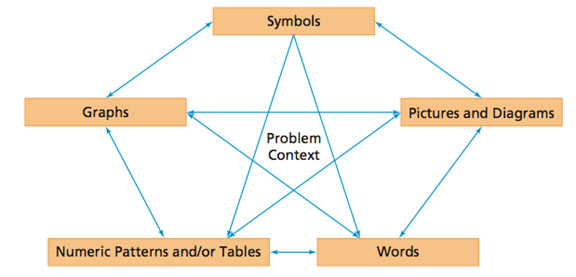#### Table

 X A 0 1 2 3 4 5 6 0 5 8 9 8 5 0

#### Graph

Note that in the graph below, x values represent the width, and y values represent the area.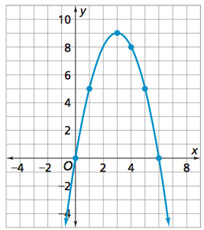#### Symbol

A = x(6-x) which was rewritten as y=x(6-x) for the graph above.

The perimeter of a rectangle is equal to twice the width plus twice the length. So, when the perimeter is 12 meters, the width plus the length must be 6 meters. This means that the maximum width is 6 meters, which would make the length 0 meters. The area would be equal to zero square meters.

These values correspond to the values in the table. Students may draw various rectangles and make a table of values for widths from 0 to 6 meters, noticing that this is the limit of the values for the width. They may also notice that the maximum area occurs when the width equals the length. In this case, the maximum area of9 square meters occurs when each dimension, the width and the length, is 3 meters.

Students may also explore rational numbers. For example, they may consider a width of 5.9 meters and a length of 0.1 meters, which results in an area of 0.59 square meters.

Students typically need some guidance to understand the difference between the domain and range of the problem situation, and the domain and range of the function used to model that problem situation. For instance, in this case, the values of the width must be between zero and 6 meters. The area must be between zero and 9 square meters. The domain of the function A = x (6 - x) is not limited, however, to positive values for x. Thus, x can be any real number. Similarly, the values for the range may be negative, with a maximum of 9.

### Example 2

Relationships between numeric equations may also be communicated using these five representations. Consider another example: the case of multiplying two mixed numbers, 51/2 x 43/4 = 261/8.

#### Words

Jane is tiling her bathroom floor. The floor is 51/2 feet long by 43/4 feet wide. What is the area of the floor that Jane is tiling? If each tile is one square foot, how many tiles does Jane need to buy?

#### Diagram

An area model fits this context nicely.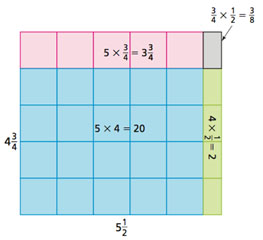#### Numeric Pattern

Students who understand multiplication as repeated addition may add 51/2 four times and then add 3/4 of 51/2, or 22 + 41/8 = 261/8

#### Graph

The problem can also be represented on a number line as repeated addition.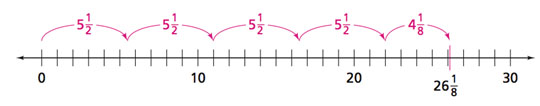### Symbols

The equation 5½  x 4¾ = 26 1/8  can be illustrated in ways that correspond 248 to the solution methods student choose.

For instance, the area model corresponds with the distributive property: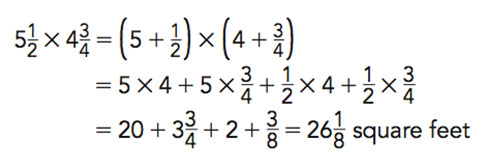So, Jane will need to purchase 27 or more tiles to tile her bathroom floor. More specifically, if Jane is accurate in cutting and fitting the tiles, she would likely need a minimum of 22 tiles plus 6 more tiles for the last row for a total of 28 tiles.

## Using Talk Moves

Use talk moves to facilitate classroom discussions. Here are the talk moves described in the work by Chapin, O’Connor, and Anderson, 2003.

• Prompt Teachers prompt students to answer a question.
• Wait Time Teachers provide adequate time for students to respond to a question.
• Revoice Teachers repeat a student’s answer to emphasize and clarify what the student said.
• Restate Teachers ask a student to restate what another student has said to ensure that students listen closely to each other.
• Apply Reasoning Teachers ask students to evaluate, critique, and use each other’s responses and strategies.

A professional development resource for facilitating effective and mathematically productive classroom discussions is the Mathematics Discourse in Secondary Classrooms (MDISC) project (Herbel-Eisenman, Steele, and Cirillo, 2013) 

### References

 Margaret S. Smith and May Kay Stein, Five Practices for Orchestrating Productive Mathematics Discussions. NCTM. 2011

 Preston, R. V., & Garner, A. S. (2003). Representation as a vehicle for solving and communication. Mathematics Teaching in the Middle School, 9, 38-43.

 Chapin, S., O’Connor, C., & Anderson, N. (2013). Classroom Discussions In Math: A Teacher's Guide for Using Talk  Moves to Support the Common Core and More, Grades K-6: A Multimedia Professional Learning Resource (third edition). Sausalito, CA: Math Solutions Publications.

 Herbel-Eisenmann, B., Steele, M., & Cirillo, M. (2013). (Developing) teacher discourse moves: A framework for professional development.Mathematics Teacher Educator, 1(2), 181-196.